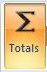## Saturday, 25 June 2011

### Using Access Queries to Produce Summary Data

In this post we shall be looking at how to use Access Queries to produce summary data on groups of records which share something in common.  The exercise which we are going to use to illustrate this involves working on a table of Order Details.  The query we are going to create will group together all the individual records which share a common value in the OrderId field, and then produce a SUM of the Amount's field for each group.  What we are effectively doing here is sorting all the records into groups of Orders and then producing a Total Amount for each individual order.  This is the table data we are going to be working with:Figure 1: The Order Details Table.
To do this exercise we are going to use GROUP BY and SUM on the TOTALS row of the QUERY DESIGN GRID.

Summary Query Data Exercise

Before creating the Query, you will first need to create the table or download the Summary_Data.accdb database.  This consists of one Order Details table with three fields - OrderId (Number), ProductId (Number), and Amount (Currency).  The primary key is a composite of OrderId and ProductId.  If this was a full Access Database Application the tblOrderDetails would be a junction table between an Orders table and a Products table. However, for our purposes of this exercise we only need to use the Order Details table.  Once this is in place you can begin the exercise.
1. Click the QUERY DESIGN icon.  This is located in the OTHER group of the CREATE RIBBON.
2. Add tblOrderDetails to the Query Design Window from the SHOW TABLE dialogue box.
3. Add the OrderId and Amount fields to the Query Design Grid.  The quickest way to do this is to double click the two field names from the Table Diagram in the top section of the Query Design Window.
4. We now need to show the TOTAL's row in the Query Design Grid.  This is not shown by default so click the TOTAL's icon in the SHOW/HIDE group of the DESIGN ribbon.  The TOTAL's row should now appear in the grid.5. We want our query to group all the records into individual orders, and calculate the total amount for each one.  This is where the TOTALS row comes in.  We are going to enter the GROUP BY clause in the TOTAL's row of the OrderId column.  (NB This is actually the default setting, so you should not need to change it). All records sharing the same OrderId will now become a seperate and distinct group (ie row) in our query results. Then move over to the TOTAL's row of the Amount column.  We are going to enter the SUM function into this cell.  You can type this in directly or select it from the drop down list.  This will give us the Sum of Amount's for each individual group in the query results.Figure 2: GROUP BY and SUM  entered into the TOTAL  row.

We can now run our query by clicking the RUN query or DATASHEET icon. Figure 3 below shows what our query results should look like:Figure 3: Query results showing a summary of our original data.

As you can see, our original records have been grouped into individual rows by their OrderId, and a sum of amount has been produced for each group/order.

#### 1 comment:

1.How do I sum two columns in Access 2007
I have one column A with numbers. I have anothe column B with another number. I need to add the two and end with the sum of the two
A+B=C